## Week 2 Coding Lecture 1: For loops

In this lecture we will discuss loops: A programming concept that allows you to repeat a block of code many times.
Suppose that we want to make the vector x = [1 2 3 4 5]. We know at least two very easy ways to make this vector: We could write it out like this
x = [1 2 3 4 5];
or we could use the colon operator like this
x = 1:5;
But suppose that we did not know the shortcut and did not want to type out the whole vector. (I will mostly stick to short vectors in these notes because they don't fill the entire command window, but you can imagine why we wouldn't want to type out the entire vector if there were 1,000 or 1,000,000 entries instead of 5.) Another approach would be to make an "empty vector" x and then fill in the correct entries. By "empty vector" I mean a vector of the appropriate size but without the correct entries.
There are a few commands in MATLAB to construct matrices and vectors. Two of the most useful are zeros and ones. As with every MATLAB function you meet, you should look at the documentation for these functions with doc zeros and doc ones. The syntax for these functions is fairly straightforward. You can use zeros(m, n) to make an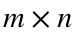matrix of all zeros or ones(m, n) to make anmatrix of all ones. Remember that a row vector is just a matrix with one row and a column vector is just a matrix with one column. If we want to make an empty vector of the same size as x, we can therefore use
x = zeros(1, 5);
(A common mistake is to try x = zeros(5) instead. This actually makes a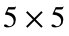matrix instead of a vector.)
Now that we have an empty vector to work with, we can fill in the entries we want. In particular, we can use
x(1) = 1;
x(2) = 2;
x(3) = 3;
x(4) = 4;
x(5) = 5;
We have now created exactly the same vector as in the previous section, albeit with much more typing. The step where we make an empty vector is called "initialization", and we say that we "initialized" the vector x. This tells MATLAB to set aside a block of memory large enough to hold a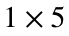vector.
It is worth noting that you can often get away without initializing your vectors in MATLAB, because MATLAB will add space to vectors as needed. For instance, you could have just used the code
x(1) = 1;
x(2) = 2;
x(3) = 3;
x(4) = 4;
x(5) = 5;
without any initialization. At each line, MATLAB adds enough space to the vector to fit the next number. However, it is worth getting into the habit of initializing vectors whenever possible. Not only will this avoid needless errors, but it is usually quite a bit faster.
As you might imagine, code like that in the above block is not very practical. This is already a tedious amount of typing with only 5 entries and would be much worse with 1,000 or 1,000,000. In addition, it is easy to make mistakes with this much code and harder to hunt them down if we find out later that we made the wrong vector. It is also tedious to make changes to this code. If we later decide that each entry of x should be twice as big, we would have to change 5 lines of code (or 1,000 or 1,000,000 if our vector were larger).
However, these five lines are almost exactly the same. This suggests that we should be able to avoid most of this typing. If we could tell MATLAB the pattern for one of these lines, then we could hopefully just tell MATLAB to repeat the pattern five times.
This is exactly what a loop is for. If you want to repeat the same block of code several times, you can use something called a "for loop". For example, to repeat some code five times, we could write the code
for k = 1:5
% Some code to be repeated
end
The words for and end are reserved words that tell Matlab this is a for loop. The variable k is called a "loop counter" or a "loop index" or a "loop variable", depending on context. You can think of it as keeping track of what step you are on. When you run this code, it will behave exactly as if you had instead typed
k = 1;
% Some code to be repeated
k = 2;
% Some code to be repeated
k = 3;
% Some code to be repeated
k = 4;
% Some code to be repeated
k = 5;
% Some code to be repeated
As you can see, the code is repeated five times and the variable k tells us which step we are on. For a particularly silly example, the following code prints the number 8 ten times:
for k = 1:10
disp(8);
end
8 8 8 8 8 8 8 8 8 8
We can also use the loop variable in the code to be repeated. This is useful for making the code do slightly different things each time. As another example, the following code prints out the first four perfect squares:
for k = 1:4
disp(k^2);
end
1 4 9 16
There is nothing special about the name k. You can call your loop index whatever you want. I will usually stick to things like i, j, k, m and n because those are common index variables in mathematics, but some people prefer more descriptive names like counter or index or loop_var. This is really just a matter of taste.
It is worth noting that MATLAB automatically indents code in the for loop for you, but the indentation is just there for readability. The keywords for and end are what actually tells MATLAB which code belongs to the loop. This is different from python, where the indentation is actually required.
Getting back to our previous example, after initializing x we want to repeat the code x(some_number) = some_number over and over again. The value of some_number starts at 1, then increases to 2, then 3, then 4, then 5. This is exactly what our loop counter did. We can rewrite our original code as
x = zeros(1, 5);
for j = 1:5
x(j) = j;
end
Remember, what MATLAB is really doing when we run this is
x = zeros(1, 5);
j = 1;
x(j) = j;
j = 2;
x(j) = j;
j = 3;
x(j) = j;
j = 4;
x(j) = j;
j = 5;
x(j) = j;
which is exactly what we wanted.
This is an incredibly common coding construct, and we will use it many times in this course. First, you initialize a variable that will be used for storing your results. Next, you use a loop to make many related calculations. The result of each of these calculations gets stored in one of the entries of your original variable. In our case, the "calculation" was just finding the value of j, but in real problems it will often be substantially more complicated.
As another example, suppose that we wanted to create the vector x = [1; 1.1; 1.2; 1.3; 1.4]. Of course, we already know a shortcut with the colon operator, but suppose that we didn't have this shortcut. We could do almost the same thing as before. (Notice that x is a column vector this time.)
x = zeros(5, 1);
x(1) = 1;
x(2) = 1.1;
x(3) = 1.2;
x(4) = 1.3;
x(5) = 1.4;
Once again, we are repeating almost the same line of code five times, so we should be able to use a loop to make this simpler. However, this problem has an extra wrinkle because there are two different numbers that change in each line: The index of x (which takes the values 1, 2, 3, 4 and 5) and the value being assigned (which takes the values 1, 1.1, 1.2, 1.3 and 1.4). A loop only keeps track of one loop variable for us. This means that we will need to deal with the other value on our own. Probably the easiest way to approach this problem is to write something like
x = zeros(5, 1);
for k = 1:5
x(k) = % Some value
end
This means that the loop will keep track of the index of x (we get to use k as the index), but we will still have to figure out what "some value" should be at each step. A standard way to do this is to start by defining a variable as whichever value we want in the first step, then incrementing this variable at every step of the loop. That is,
x = zeros(5, 1);
x_value = 1;
for k = 1:5
x(k) = x_value;
x_value = x_value + 1;
end
The line x_value = 1 is also called "initialization". Here we are initializing the variable x_value. The line x_value = x_value + 0.1 increments this value by 0.1 after each step. The first time through the loop, x_value is 1 (as desired). The second time through the loop it is 1.1, then 1.2, then 1.3, then 1.4.
This is another very common pattern in programming. If you have a variable that changes at each step in a loop, you can initialize it to some useful starting value, then update it at the end of each loop step.
The incrementing approach described above is a standard approach and should almost always be your first choice. In this case, however, we have another interesting option: We can find a formula for x_value in terms of k. In particular, we can use
x = zeros(5, 1);
for k = 1:5
x(k) = 1 + 0.1 * (k - 1);
end
You should convince yourself that this makes exactly the same vector as in the previous example.
It is not always easy to find a formula like this, but if you happen to notice one then this is often a good approach. It tends to be much harder to think up and possibly a little bit slower, but often doesn't accumulate as much rounding error. As with many things, unless you are extremely concerned about efficiency, which method you choose is largely a matter of taste.
It is worth noting that the line k = 1:5 does something slightly different than the line for k = 1:5. The former produces a vector named k with the value [1 2 3 4 5], but the latter produces a variable k that is only ever a single number at a time. This is easy to forget but quite important.
It is also worth noting that there is nothing particularly special about the colon syntax in a for loop. You can actually write for k = vec where vec is any row vector. (In fact, vec can also be a column vector or matrix, but the loop behaves somewhat differently - we will never use that syntax in this class.) For example, we could write
for n = [1 3 -10 2.5]
disp(n);
end
1 3 -10 2.5000
This is equivalent to writing
n = 1;
disp(n);
1
n = 3;
disp(n);
3
n = -10;
disp(n);
-10
n = 2.5;
disp(n);
2.5000
We will almost always use the colon syntax, but it is occasionally useful to be able to loop over other row vectors.

### Nested loops

Now let's try something slightly more complicated. Instead of filling out the entries of a vector, we will fill out the entries of a matrix. For starters, we will try to create the matrix A = [1 1 1; 2 2 2; 3 3 3; 4 4 4].
If we do not want to type the whole matrix out (and once the matrix gets large that would be very impractical) then we can use a similar approach to the last section. For instance, we could type
A = zeros(4, 3);
A(1, :) = 1;
A(2, :) = 2;
A(3, :) = 3;
A(4, :) = 4;
This initializes the matrix (creates a zero matrix of the right size), then sets each row in turn. As in our previous examples, we have almost exactly repeated the same line of code several times, which means that this is a prime candidate for a loop. Following the same logic as above, we can write
A = zeros(4, 3);
for k = 1:4
A(k, :) = k;
end
This produces the desired matrix.
Now suppose that we want to make the matrix A = [1 2 3; 4 5 6; 7 8 9; 10 11 12]. Following a similar strategy, we could initialize our matrix and then fill in each value one at a time:
A = zeros(4, 3);
A(1, 1) = 1;
A(1, 2) = 2;
A(1, 3) = 3;
A(2, 1) = 4;
A(2, 2) = 5;
A(2, 3) = 6;
A(3, 1) = 7;
A(3, 2) = 8;
A(3, 3) = 9;
A(4, 1) = 10;
A(4, 2) = 11;
A(4, 3) = 12;
Once again, this seems like a good candidate for a loop, but this time we have three values that are changing: The row number, the column number and the value on the right hand side. One approach might be to have one loop for k = 1:12 and perform each of these 12 steps, but it would end up being pretty messy to keep track of the necessary variables. A better method is to think of each block of three commands as a single piece of code. We really repeat each of those blocks three times. This means that we want something like
A = zeros(4, 3);
for i = 1:4
A(i, 1) = % Some value
A(i, 2) = % Some other value
A(i, 3) = % Some different value
end
Look at the three lines inside this for loop: They are really just the same line of code repeated three times with slightly different numbers, which makes them another good candidate for a for loop. We can therefore write
A = zeros(4, 3);
for i = 1:4
for j = 1:3
A(i, j) = % Some value
end
end
Now all we need to do is keep track of "some value". Here is one of the more straightforward methods (very similar to one of the examples from the single for loops section):
A = zeros(4, 3);
A_value = 1;
for i = 1:4
for j = 1:3
A(i, j) = A_value;
A_value = A_value + 1;
end
end
You should carefully study this example until you can clearly see why it creates the matrix we are looking for and what order everything happens in. In particular, it may help to have MATLAB print out the values of i, j and A_value at every step.
As you can see, code inside the for loop does not have to be just one line; it can be arbitrarilly complicated. In particular, we are allowed to put loops inside other loops. These are called nested for loops. The loop involving i (the one where the for and end are not indented) is called the outer loop. The loop involving j (the one where the for and end are indented once) is called the inner loop. We will often need two loops nested together like this example, but it is probably wise to avoid going any deeper than this. MATLAB doesn't have any limit on how many loops you can nest (well, it does, but the limit is very very large), but you will find that your code becomes both very slow and very hard to understand once you have too many nested loops.

## Fibonacci numbers

Now let's try a more interesting mathematical example. We will try to calculate some Fibonacci numbers. The Fibonacci numbers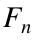are defined by the following recurrence relation: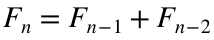,
where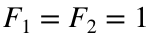. That is, the nth Fibonacci number is the sum of the previous two. We will start by calculating the first 20 Fibonacci numbers and saving them in a vector. This is the first example where we really do need a loop: There is no MATLAB shortcut like the colon operator for calculating Fibonacci numbers (well, there is actually a builtin function in one of the toolboxes, but we will ignore it).
We will use the same basic approach as before: Initialize a vector for our Fibonacci numbers, then use a loop to fill in each entry of the vector in turn. The basic skeleton is
fib = zeros(1, 20);
for n = % something
fib(n) = % calculate the nth fibonacci number
end
There are quite a few variations we could use to write this code, but probably the most straightforward way is to essentially copy the definition into our loop like so:
fib = zeros(1, 20);
fib(1) = 1;
fib(2) = 1;
for n = % something
fib(n) = fib(n - 1) + fib(n - 2);
end
Notice that we included the first two Fibonacci numbers in the initialization step. This is just part of the definition of the problem. We can now figure out what the loop variable is supposed to be. We have already filled out the first two values, so n should start at 3, and we want to fill out everything up to the 20th entry, so n should end at 20. We therefore have
fib = zeros(1, 20);
fib(1) = 1;
fib(2) = 1;
for n = 3:20
fib(n) = fib(n - 1) + fib(n - 2);
end
Suppose that we decide to calculate the first 200 numbers instead. We can use almost exactly the same code, but we have to make two changes: We need to change the loop variable from n = 3:20 to n = 3:200 and we need to change the initialization line so that fib is the right size.
fib = zeros(1, 200);
fib(1) = 1;
fib(2) = 1;
for n = 3:200
fib(n) = fib(n - 1) + fib(n - 2);
end
It is pretty easy to forget one of these changes. (If you forget to change the zeros command, your code will still work, but will run slower. If you forget to change the loop bounds then your code won't work correctly.) Since both numbers are always supposed to be the same, it is a good idea to make a variable for the number of Fibonacci numbers that we are calculating.
total_numbers = 200;
fib = zeros(1, total_numbers);
fib(1) = 1;
fib(2) = 1;
for n = 3:total_numbers
fib(n) = fib(n - 1) + fib(n - 2);
end
This way we only need to change the value of total_numbers and the rest of our code will work as desired.
We have successfully calculated the first 20 (or 200, or any number) Fibonacci numbers. In fact, this code is pretty close to the best solution we can get for this problem. However, imagine that we modify the problem slightly. Suppose that instead of the first 20 numbers, we want to find all the Fibonacci numbers that are less than 1,000,000. Now our solution doesn't work. The issue is that we don't know beforehand how many numbers we will need, so we don't know what our loop variable should be.
To solve this problem, we want to start calculating Fibonacci numbers like before, but tell MATLAB to stop when fib(n) reaches 1,000,000. To write this in code, we will need a new concept that tells MATLAB "if fib(n) is bigger than 1,000,000, stop the loop". We will talk about this concept in the next lecture.